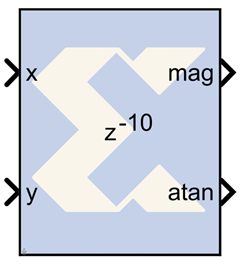# CORDIC ATAN - 2020.2 English

## Vivado Design Suite Reference Guide: Model-Based DSP Design Using System Generator (UG958)

Document ID
UG958
Release Date
2020-11-18
Version
2020.2 English

The Xilinx CORDIC ATAN reference block implements a rectangular-to-polar coordinate conversion using a fully parallel CORDIC (COordinate Rotation DIgital Computer) algorithm in Circular Vectoring mode.That is, given a complex-input <x,y>, it computes a new vector <m,a>, where magnitude m = K x sqrt (x2 + y2), and the angle a = arctan(y/x). As is common, the magnitude scale factor K = 1.646760... is not compensated in the processor, for example, the magnitude output should be scaled by this factor. The CORDIC processor is implemented using building blocks from the Xilinx blockset.

The CORDIC ATAN algorithm is implemented in the following 3 steps:

1. Coarse Angle Rotation. The algorithm converges only for angles between -pi/2 and pi/2, so if x < zero, the input vector is reflected to the 1st or 3rd quadrant by making the x-coordinate non-negative.
2. Fine Angle Rotation. For rectangular-to-polar conversion, the resulting vector is rotated through progressively smaller angles, such that y goes to zero. In the i-th stage, the angular rotation is by either +/- atan(1/2i), depending on whether or not its input y is less than or greater than zero.
3. Angle Correction. If there was a reflection applied in Step 1, this step applies the appropriate angle correction by subtracting it from +/- pi.

## Block Parameters

The block parameters dialog box can be invoked by double-clicking the icon in your Simulink model.

Parameters specific to this reference block are as follows:

• Number of Processing Elements: specifies the number of iterative stages used for fine angle rotation.
• X,Y Data Width: specifies the width of the inputs x and y. The inputs x, and y should be signed data type having the same data width.
• X,Y Binary Point Position: specifies the binary point position for inputs x and y. The inputs x and y should be signed data type with the same binary point position.
• Latency for each Processing element: This parameter sets the pipeline latency after each circular rotation stage.

The latency of the CORDIC arc tangent block is calculated based on the formula specified as follows: Latency = 3 + sum (latency of Processing Elements)

## Reference

1) J. E. Volder, The CORDIC Trigonometric Computing Technique, IRE Trans. On Electronic Computers, Vol. EC-8, 1959, pp. 330-334.

2) J. S. Walther, A Unified Algorithm for Elementary Functions, Spring Joint Computer Conference (1971) pp. 379-385.

3) Yu Hen Hu, CORDIC-Based VLSI Architectures for Digital Signal Processing, IEEE Signal Processing Magazine, pp. 17-34, July 1992.